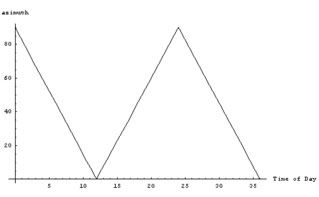# The Flat Sky

• 2 Replies
• 1022 Views##### The Flat Sky
« on: January 15, 2007, 09:41:27 PM »
I thought this would be pretty interesting, but I don't know if anyone else has done this before because the search doesn't work too great.

What follows is what the FE model sun would look like standing on the Equator over a 36 hour period.

Assumptions:
Sun is 3000 miles above Earth equator.
Radius of equator is 25000 / 4 = 6250 miles.
Sun is at its average radius.
Observer is looking north.

Use parametric equation of a circle so position of sun is based on time of day:
x=r*cos(t * pi/12)
y=r*sin(t * pi/12)

Use Distance formula to find distance from observer to sun at any time.

Use right triangle to find inclination of sun at any time: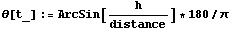now plot theta with respect to time of day: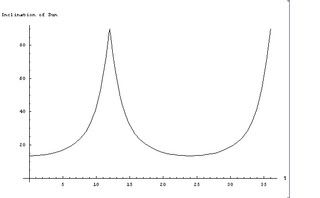The peaks at 90 degrees indicate when the sun is directly overhead (12 hours), and the minimum is midnight.  As you can see from the graph, the sun is still 14 degrees above the horizon at midnight.

The apparent speed of the sun through the sky can be found from the sum of the derivatives of the spherical coordinates theta (from above) and phi: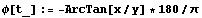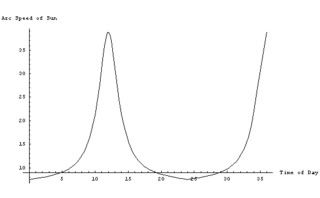I tried to get mathematica to make a pretty spherical plot of all this but it was complaining too much.

There needs to be a new FE model.  Someone should come up with some constants for the MOND that would create enough lensing for the sun to set (or would that crush us?).  Or figure out how the pressure gradient of the atmosphere effects the index of refraction.

And again, if I screwed anything up just tell me and I'll recalculate.##### The Flat Sky
« Reply #1 on: January 16, 2007, 11:56:50 AM »
If there were a large enough index of refraction to make the sun actually set, the sun would change colors as it approached the horizon.##### The Flat Sky
« Reply #2 on: January 17, 2007, 02:13:34 PM »
For reference, here is the plot of the azimuth angle phi, with respect to the equator: